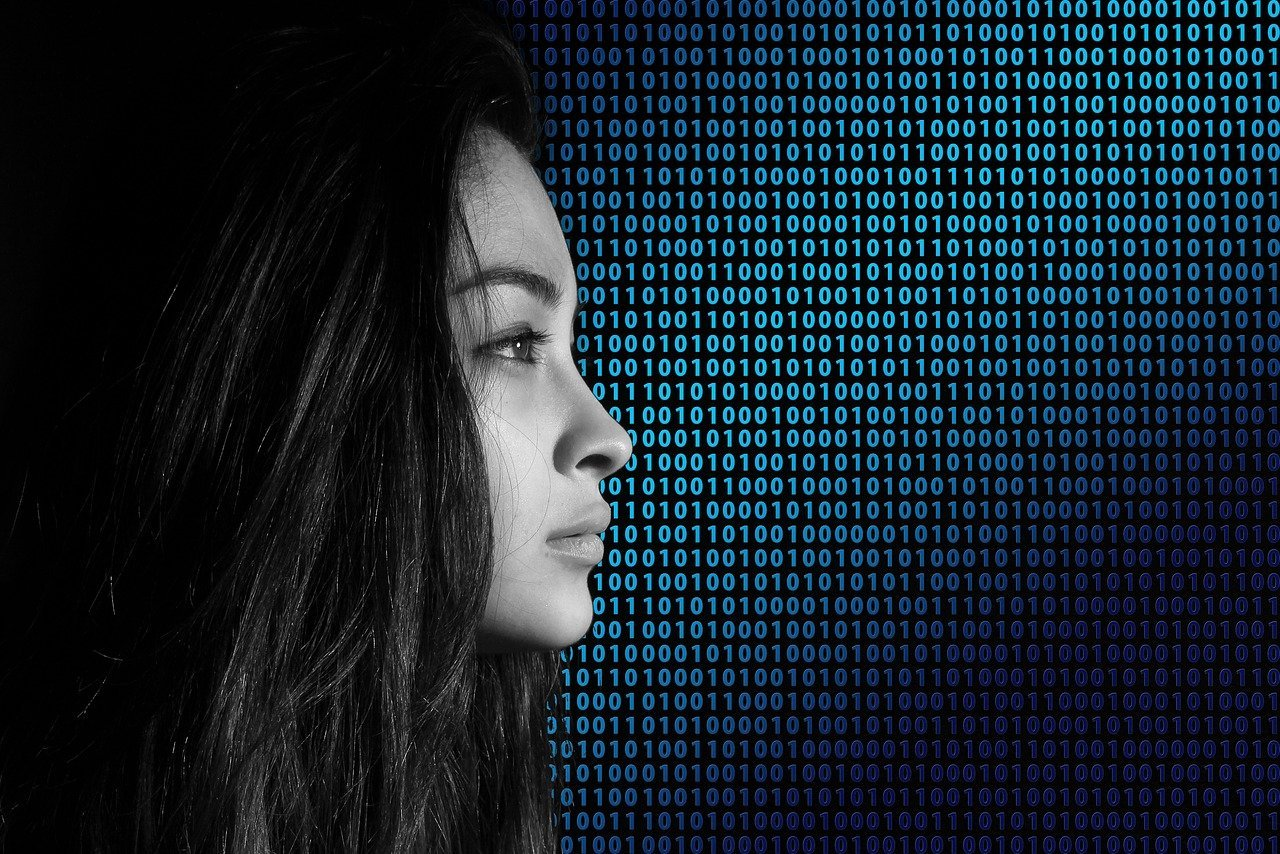Connect with us

# Binary to Text: When ‘I Love You’ Becomes ‘01101000-ly’Published

onIn today’s digital age, the binary code serves as the foundation of all computer systems and data storage. This intricate language of ones and zeros underpins every aspect of the digital world, from software and hardware to the internet itself. Yet, to the uninitiated, binary code can appear as cryptic as an ancient manuscript.

Let us try to demystify the world of binary code, explaining what it is, how to convert it into human-readable text, and answering common questions.

## Understanding Binary Code

Binary code is a numerical system that employs only two symbols, typically represented as 0 and 1. These symbols correspond to the absence (0) or presence (1) of an electrical voltage in the context of computer hardware. Every piece of data in the digital realm, whether it’s a text document, image, or software program, can be translated into binary code for processing by computers.

## Converting Binary to Text

Converting binary code into human-readable text is a straightforward process. Each group of eight binary digits, or bits, represents a single character through an encoding scheme called ASCII (American Standard Code for Information Interchange). To decode binary into text, follow these steps:

Break It Down: Split the binary code into groups of eight bits each. These eight-bit groups are called bytes and represent one character.

Convert to Decimal: Convert each byte from binary to decimal. This is done by multiplying each bit’s position (from right to left) by 2 raised to the power of its position. For example, in the binary number 01101000, the rightmost bit represents 2^0 (1), the next bit to the left represents 2^1 (2), and so on.

Reference ASCII: Use an ASCII table to find the corresponding character for each decimal value. ASCII assigns each character a unique decimal value, making it possible to convert binary into text.

Repeat for Each Byte: Continue this process for every byte in the binary code.

## What Is the Binary Code for ‘I Love You’?To uncover the binary code for the heartfelt phrase “I Love You,” we first need to determine the ASCII values for each letter. Here’s a breakdown:

– ‘I’ corresponds to 73 in decimal (01001001 in binary).
– ‘Space’ between words corresponds to 32 in decimal (00100000 in binary).
– ‘L’ corresponds to 76 in decimal (01001100 in binary).
– ‘o’ corresponds to 111 in decimal (01101111 in binary).
– ‘v’ corresponds to 118 in decimal (01110110 in binary).
– ‘e’ corresponds to 101 in decimal (01100101 in binary).
– ‘Space’ corresponds to 32 in decimal (00100000 in binary).
– ‘Y’ corresponds to 89 in decimal (01011001 in binary).
– ‘o’ corresponds to 111 in decimal (01101111 in binary).
– ‘u’ corresponds to 117 in decimal (01110101 in binary).

Putting it all together, “I Love You” in binary code looks like this:

01001001 00100000 01001100 01101111 01110110 01100101 00100000 01011001 01101111 01110101

## Binary Code Explained: Common Queries

Let’s address some common queries related to binary code:

### What Is the Binary Code 011101?

Binary code is often grouped into sets of eight bits or one byte to represent a character or value. The binary code 011101, however, is incomplete, as it consists of only six bits. A complete byte is necessary to translate binary into a corresponding character using ASCII encoding.

### What Does 01010011 Mean in Binary?

The binary code 01010011 corresponds to the decimal value 83, which, in ASCII, represents the character ‘S’. So, in binary code, 01010011 is equivalent to the letter ‘S’.

### What Does 01110011 Mean in Binary?

The binary code 01110011 translates to the decimal value 115, which corresponds to the character ‘s’ in ASCII. Therefore, in binary code, 01110011 stands for the lowercase letter ‘s’.

### What Is 111 in Binary?

The binary code 111 represents the decimal value 7. In ASCII, 7 does not correspond to a traditional character but rather represents an end-of-text signal, used in some data transmission systems.

## Binary to Text Code 01010101

Binary code 01010101 translates to the decimal value 85, which, in ASCII, represents the character ‘U’. Therefore, in binary, 01010101 stands for the uppercase letter ‘U’.

## Conclusion: Deciphering the Binary to Text

Binary code, with its intricate dance of ones and zeros, is the language of the digital age. Whether you’re an aspiring coder or simply curious about the inner workings of your computer, understanding binary code is a valuable skill. With the guidance provided in this article, you can now convert binary into human-readable text, decode secret messages, or simply appreciate the foundational role binary plays in our technology-driven world. In a realm where every bit counts, you’re well on your way to becoming a binary bard!Click to comment